• 文章目录1、安装库2、xlsxwriter写入Excel3、openpyxl写入Excel4、写入有格式的excel表 1、安装库 打开cmd命令行输入命令pip install xlsxwriter 和 pip install openpyxl即可安装 xlsxwriter文档 openpyxl中文文档...


文章目录
1、安装库2、xlsxwriter写入Excel3、openpyxl写入Excel4、写入有格式的excel表

1、安装库
打开cmd命令行输入命令pip install xlsxwriter 和 pip install openpyxl即可安装
xlsxwriter文档
openpyxl中文文档

2、xlsxwriter写入Excel
import xlsxwriter as xw

#指定excel路径，没有就创建
work = xw.Workbook("G:/PyCharmProjects/PythonAutomation/excel/newtest01.xlsx")

#新建工作表

for i in range(0,301):
sheet.write(0,i,i)

#关闭
work.close()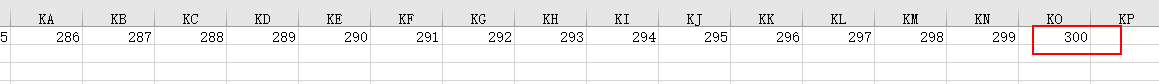3、openpyxl写入Excel
import openpyxl

sheet = work['one']
sheet['A2'] = "A2"
sheet['A3'] = "A3"
sheet['A4'] = "A4"
sheet['A5'] = "A5"

work.save("G:/PyCharmProjects/PythonAutomation/excel/newtest01.xlsx")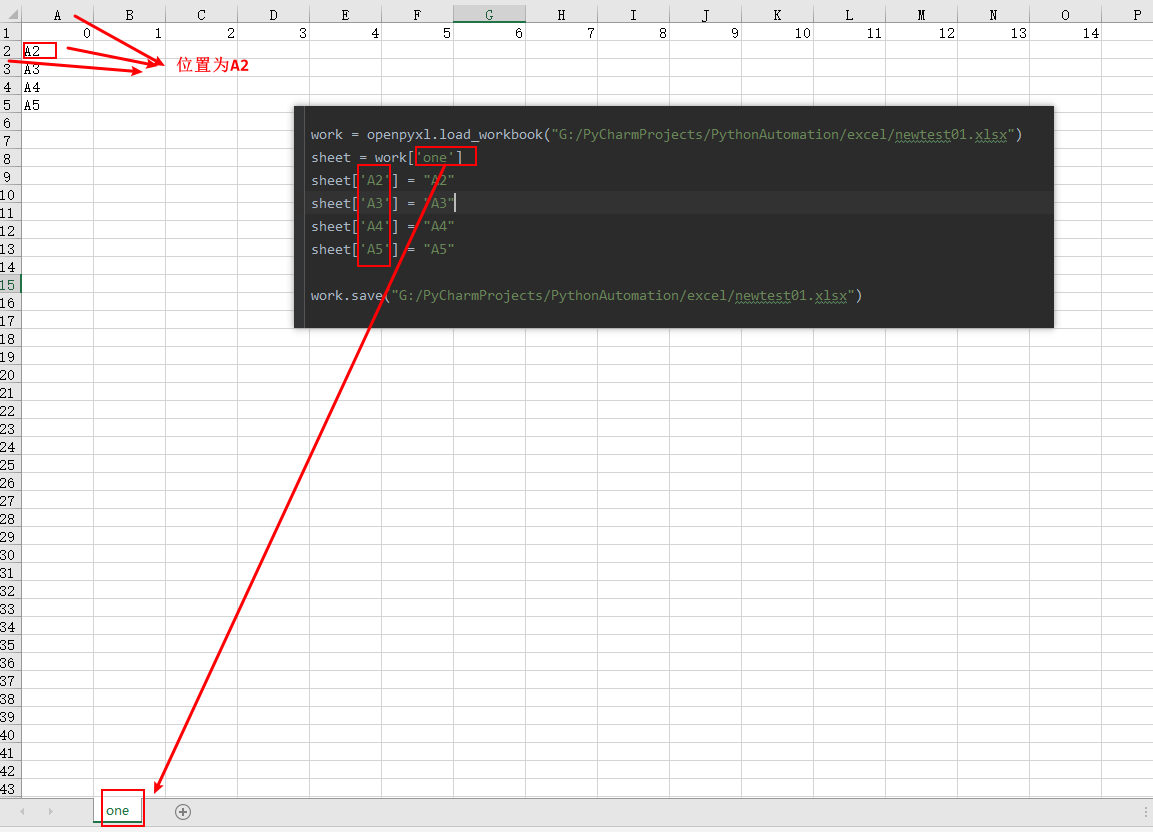4、写入有格式的excel表
新建有样式的excel表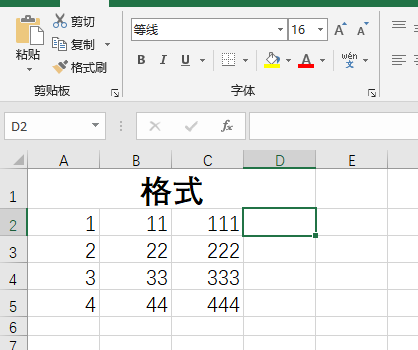使用xlsxwriter写入
import xlsxwriter as xw

work = xw.Workbook("G:/PyCharmProjects/PythonAutomation/excel/geshi.xlsx")

for i in range(1,5):
#指定第i行，第3列写入（索引从0开始）
sheet.write(i,3,i)
work.close()


原有数据丢失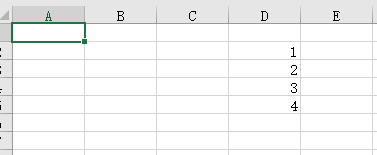使用openpyxl写入
import openpyxl

#指定工作表
sheet = work['Sheet1']
#写入
sheet['A2'] = "A2"
sheet['A3'] = "A3"
sheet['A4'] = "A4"
sheet['A5'] = "A5"

work.save("G:/PyCharmProjects/PythonAutomation/excel/geshi.xlsx")


可见，样式没有问题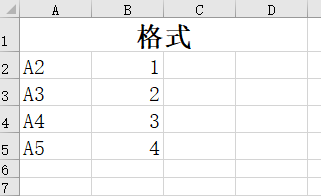博主会持续更新，有兴趣的小伙伴可以点赞、关注和收藏下哦，你们的支持就是我创作最大的动力！
Java学习从入门到大神学习目录索引
博主开源Python爬虫教程目录索引（宝藏教程，你值得拥有！）
Python自动化办公专栏展开全文python 自动化办公 教学
• 写入EXCEL文件 from openpyxl import Workbook a = "aaaaaaaa" b = "bbbbbbbb" c = "cccccccc" d = "dddddddd" e = "eeeeeeee" f = "ffffffff" wb = Workbook() ws = wb.active tableTitle = [a, b,c,] for col in ...
写入EXCEL文件
from openpyxl import Workbook

a = "aaaaaaaa"
b = "bbbbbbbb"
c = "cccccccc"
d = "dddddddd"
e = "eeeeeeee"
f = "ffffffff"
wb = Workbook()
ws = wb.active
tableTitle = [a, b,c,]
for col in range(len(tableTitle)):
c = col + 1
ws.cell(row=1, column=c).value = tableTitle[col]
# 数据表基本信息
tableValues = [[d, e, f,]]
for row in range(len(tableValues)):
ws.append(tableValues[row])
# wb.save(ExcelFullName)
wb.save("d:\\1.xlsx")

展开全文• 报错如下： ValueError: Cannot convert to excel 原因是因为我们使用语句 wb = openpyxl.Workbook() ws1 = wb.active() ws = wb.create_sheet...原本我要写入Excel的是[[],[],[]] 格式的数据，但是由于不支持复合
报错如下：
ValueError: Cannot convert to excel
原因是因为我们使用语句
wb = openpyxl.Workbook()
ws1 = wb.active()
ws = wb.create_sheet("Sheet1", 0)

// 格式 [[],[]]
// 报错
ws.append(i)

// 正确
for i in list_result:
ws.append(i)
原本我要写入Excel的是[[],[],[]] 格式的数据，但是由于不支持复合集合，所以就报错了
ValueError: Cannot convert to excel
因此，要不然就拆分单个字符，使用str()转化为字符串或者就是弄成单个列表。
展开全文Python
• openpyxl 提供了写入 Excel 的方法，这样我们就可以通过 Python 程序来创建或编辑 Excel 文件啦O(∩_∩)O哈哈~ 1 创建与保存文件 import openpyxl # 创建 wb = openpyxl.Workbook() print('sheetnames = ' + str(wb...openpyxl 提供了写入 Excel 的方法，这样我们就可以通过 Python 程序来创建或编辑 Excel 文件啦O(∩_∩)O哈哈~
1 创建与保存文件
import openpyxl

# 创建
wb = openpyxl.Workbook()
print('sheetnames = ' + str(wb.sheetnames))

sheet = wb.active
print('sheet.title = ' + sheet.title)

sheet.title = '人口统计'

print('sheetnames = ' + str(wb.sheetnames))

# 保存
wb.save('人口.xlsx')


运行结果：

sheetnames = [‘Sheet’] sheet.title = Sheet sheetnames = [‘人口统计’]

通过 openpyxl.Workbook() 函数，就可以创建一个 Workbook 对象。调用 save() 方法，就可以保存 Workbook 对象为 Excel 文件。
也可以加载某份 Excel 文件之后，将其内容保存在另一份文件中：
wb = openpyxl.load_workbook('人口.xlsx')
sheet = wb.active
sheet.title = '地区'
wb.save('地区.xlsx')

注意： 当我们加载并修改了某份 Excel 文件之后，建议将其保存到另一份 Excel 文件中。这样做有以下好处：
如果代码存在缺陷，还有修复挽回的余地。最初的那份 Excel 文件还可以用于二次处理。
2 新建与删除工作表（ sheet）
# 创建工作表
print('开始创建工作表……')
wb.create_sheet()
print('sheetnames = ' + str(wb.sheetnames))
wb.create_sheet(index=2, title='人口分布')
print('sheetnames = ' + str(wb.sheetnames))
print('创建完成。')

# 移除工作表
print('开始移除工作表……')
del wb['Sheet']
print('sheetnames = ' + str(wb.sheetnames))
print('移除完成。')

运行结果：

开始创建工作表…… sheetnames = [‘人口统计’, ‘Sheet’] sheetnames = [‘人口统计’, ‘Sheet’, ‘人口分布’] 创建完成。 开始移除工作表…… sheetnames = [‘人口统计’, ‘人口分布’] 移除完成。

create_sheet() 可以创建工作表。其 index 与 title 参数，可以指定工作表的索引和名称。del wb[\${sheet_name}] 可以删除指定名称的工作表。
3 写入单元格
sheet = wb.active
sheet['A1'] = '中国'
print('A1 = ' + str(sheet['A1'].value))


运行结果：

A1 = 中国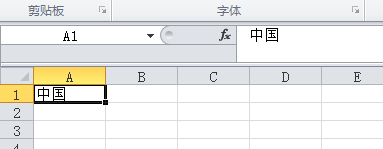展开全文Python
• 使用 xlsxwriter/pandas/openpyxl 三个库来实现数据写入 EXCEL ，以及向已存在文件追加数据python
• 如图的表格，设定的是第二列有几个数据的个数是从微信小程序前端传过来的，那么怎么根据第二列的个数来合并第一列呢，并且自动写入从前端传过来的例如阳台，橱柜之类的标题。 求解答，不升ganxie。python
• from openpyxl.styles import Font,Alignment,Side,Border,Color,colors,PatternFill from MMSpackagefile.codefile.ExcelData import ExcelData class CompareExcel(object): def __init__(self): pass ...python
• 小弟最近在使用 Python 读取 Excel 数据，然后写入到另一个 sheet 时，遇到个问题，想请教一下各位朋友。 详细请看这里： [color=#FF0000]环境[/color]： Python 2.7 和 [color=#FF0000]openpyxl[/color] ...python
•python
• python使用openpyxl写入、读取excel xlsx文件 openpyxl openpyxl（可读写excel表）专门处理Excel2007及以上版本产生的xlsx文件，xls和xlsx之间转换容易（**P.S.**文字编码是“gb2312” 读取后就会显示乱码，请先转成...python
• 主要介绍了python3.7 openpyxlexcel单元格中写入数据实例，具有很好的参考价值，希望对大家有所帮助。一起跟随小编过来看看吧
• import openpyxl,os,re import requests #第三方下载器 from requests import RequestException from bs4 import BeautifulSoup #定义list 存入D列 最新净值数据 list_d = [] def getExcel_D(file_url): excel...python
• openpyxlExcel写入数据后原表中的斜线丢失，请叫各位，有没有什么办法解决，或者有没有什么库可以向Excel中加入斜线的，不胜感激有问必答 python
• 利用openpyxl实现写入Excel的xlsx文件，我们先下载openpyxl模块： pip installopenpyxl 具体写入excel表格代码如下： import openpyxl # openpyxl引入模块 def write_to_excel(path: str, sheetStr, info, data)...
• 本来我是想尝试，选中某个多个单元格复制到同一个sheet的其他位置，...# encoding:utf-8import pandas as pdimport openpyxlxl = pd.read_excel(r"E:\55\CRM经营分析表-10001741-1570416265044.xls")xl.to_excel(r"E...
• 原标题：Python openpyxlExcel 文档简单操作背景：生活中常常因日常工作，在记录统计方面需频繁处理较多 Excel 表格，这部分工作虽可由人工完成，但这样会显得有些繁琐且可能存在偏差，遂闲时查阅了是否有相关...
• from openpyxl import Workbook from openpyxl.utils import get_column_letter print(range(100)) #创建一个工作表 wb = Workbook() #工作表名称变量 dest_filename = ‘empty_book.xlsx’ #Sheet页第一个...pythonpython
• 一、openpyxl模块介绍1、openpyxl是读写Excel的python库，是一个比较综合的工具，能够同时读取和修改Excel文档2、openpyxl中有三个不同层次的类,每一个类都有各自的属性和方法：Workbook是一个excel工作表Worksheet...
• from openpyxl import Workbook wb = Workbook() # grab the active worksheet ws = wb.active # Data can be assigned directly to cells ws['A1'] = 42 # Rows can also be appended ws.append([1, 2, 3])...